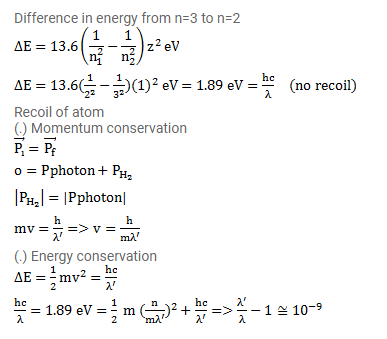# When a photon is emitted from an atom,

Question:

When a photon is emitted from an atom, the atom recoils. The kinetic energy of recoil and the energy of the photon come from the difference in energies between the states involved in the transition. Suppose, a hydrogen atom changes its state from $n=3$ to $n=2$ Calculate the fractional change in the wavelength of light emitted, due to the recoil.

Solution: